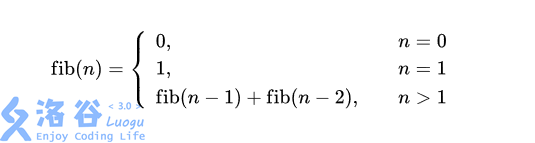# P4994 终于结束的起点

• 2.2K通过
• 7.5K提交
• 题目提供者 fstqwq 管理员
• 评测方式 云端评测
• 标签 斐波那契,Fibonacci 枚举,暴力 递推
• 难度 普及/提高-
• 时空限制 1000ms / 512MB
• 提示：收藏到任务计划后，可在首页查看。

## 题目背景

终于结束的起点
终于写下句点
终于我们告别
终于我们又回到原点
……

一个个 OIer 的竞赛生涯总是从一场 NOIp 开始，大多也在一场 NOIp 中结束，好似一次次轮回在不断上演。
如果这次 NOIp 是你的起点，那么祝你的 OI 生涯如同夏花般绚烂。
如果这次 NOIp 是你的终点，那么祝你的 OI 回忆宛若繁星般璀璨。
也许这是你最后一次在洛谷上打比赛，也许不是。
不过，无论如何，祝你在一周后的比赛里，好运。

当然，这道题也和轮回有关系。

## 题目描述

广为人知的斐波拉契数列 $\mathrm{fib}(n)$ 是这么计算的也就是 $0, 1, 1, 2, 3, 5, 8, 13 \cdots$，每一项都是前两项之和。

小 F 发现，如果把斐波拉契数列的每一项对任意大于 $1$ 的正整数 $M$ 取模的时候，数列都会产生循环。

当然，小 F 很快就明白了，因为 ($\mathrm{fib}(n - 1) \bmod M$) 和 ($\mathrm{fib}(n - 2) \bmod M)$ 最多只有 $M ^ 2$ 种取值，所以在 $M ^ 2$ 次计算后一定出现过循环。

甚至更一般地，我们可以证明，无论取什么模数 $M$，最终模 $M$ 下的斐波拉契数列都会是 $0, 1, \cdots, 0, 1, \cdots$。

现在，给你一个模数 $M$，请你求出最小的 $n > 0$，使得 $\mathrm{fib}(n) \bmod M = 0, \mathrm{fib}(n + 1) \bmod M = 1$。

## 输入输出格式

输入格式：

输入一行一个正整数 $M$。

输出格式：

输出一行一个正整数 $n$。

## 输入输出样例

输入样例#1： 复制
2
输出样例#1： 复制
3
输入样例#2： 复制
6
输出样例#2： 复制
24

## 说明

#### 样例 1 解释

斐波拉契数列为 $0, 1, 1, 2, 3, 5, 8, 13, 21, 34, \cdots$，在对 $2$ 取模后结果为 $0, 1, 1, 0, 1, 1, 0, 1, 1, 0, \cdots$。

我们可以发现，当 $n = 3$ 时，$f(n) \bmod 2= 0, f(n + 1) \bmod 2 = 1$，也就是我们要求的 $n$ 的最小值。

#### 数据范围

对于 $30\%$ 的数据，$M \leq 18$；

对于 $70\%$ 的数据，$M \leq 2018$；

对于 $100\%$ 的数据，$2 \leq M \leq 706150=$0xAC666

#### 提示

如果你还不知道什么是取模 $(\bmod)$，那我也很乐意告诉你，模运算是求整数除法得到的余数，也就是竖式除法最终「除不尽」的部分，也即 $$a \bmod M =k \iff a = bM + k\ (M > 0, 0 \leq k < M)$$ 其中 $a, b, k$ 都是非负整数。

如果你使用 C / C++，你可以使用 % 来进行模运算。

如果你使用 Pascal，你可以使用 mod 来进行模运算。

提示
标程仅供做题后或实在无思路时参考。
请自觉、自律地使用该功能并请对自己的学习负责。
如果发现恶意抄袭标程，将按照I类违反进行处理。